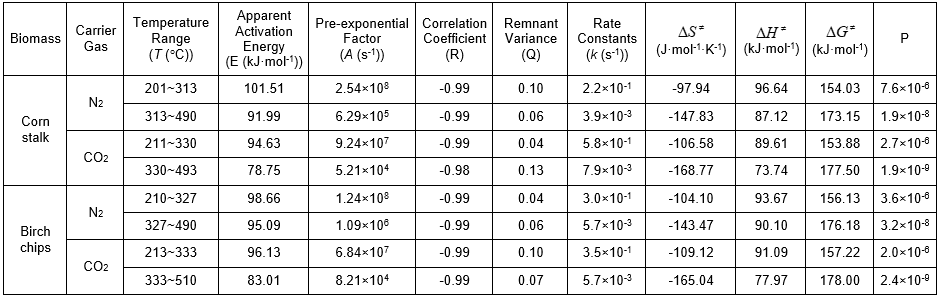Zhai, M., Guo, L., Zhang, Y., Dong, P., Qi, G., and Huang, Y. (2016). "Kinetic parameters of biomass pyrolysis by TGA," BioRes. 11(4), 8548-8557.

#### Abstract

Pyrolysis processes of typical biomass, such as corn stalk and birch chips, were investigated by thermogravimetric analysis (TGA). The apparent activation energy and pre-exponential factor were calculated with the adoption of the improved Coats-Redfern integral method, 46 types of common mechanism functions, and the least square and iterative methods. By applying basic parameters of biomass pyrolysis, a reaction rate constant, activation entropy and activation enthalpy, activation Gibbs free energy, and the steric-hindrance factor were all calculated. Results showed that biomass pyrolysis can be divided into two primary reaction zones (Event 3 and Event 4). Event 3 is focused by cellulose and hemicellulose. Event 4 is oriented by lignin and cellulose. The thermogravimetric curves of the two biomass types under carbon dioxide and nitrogen atmospheres were roughly similar. The reaction mechanism function is 1 – alpha. It is possible to use activation entropy to represent the pre-exponential factor and to use the steric hindrance factor to predict the reaction rate.

Kinetic Parameters of Biomass Pyrolysis by TGA

Ming Zhai,a,* Li Guo,a Yu Zhang,a Peng Dong,a Guoli Qi,b and Yudong Huang a

Pyrolysis processes of typical biomass, such as corn stalk and birch chips, were investigated by thermogravimetric analysis (TGA). The apparent activation energy and pre-exponential factor were calculated with the adoption of the improved Coats-Redfern integral method, 46 types of common mechanism functions, and the least square and iterative methods. By applying basic parameters of biomass pyrolysis, a reaction rate constant, activation entropy and activation enthalpy, activation Gibbs free energy, and the steric-hindrance factor were all calculated. Results showed that biomass pyrolysis can be divided into two primary reaction zones (Event 3 and Event 4). Event 3 is focused by cellulose and hemicellulose. Event 4 is oriented by lignin and cellulose. The thermogravimetric curves of the two biomass types under carbon dioxide and nitrogen atmospheres were roughly similar. The reaction mechanism function is 1 – . It is possible to use activation entropy to represent the pre-exponential factor and to use the steric hindrance factor to predict the reaction rate.

Keywords: Biomass pyrolysis; Thermogravimetric analysis (TGA); Reaction mechanism; Activation entropy; Steric hindrance factor

Contact information: a: Harbin Institute of Technology, Harbin, China, 150001; b: China Special Equipment Inspection and Research Institute, Beijing 100029;

* Corresponding author: zhaiming@hit.edu.cn

INTRODUCTION

Biomass pyrolysis is an extremely complex process with physical and chemical changes. However, the essence of the pyrolysis phenomenon is a series of complex chemical changes in which organic macromolecules rupture successively and form volatile matter and solid products at a specific temperature (Blasi 2008). The pyrolysis process can be regarded as a linear superposition of the pyrolysis processes of cellulose, hemicellulose, and lignin (Demirbas 2000). The pyrolysis processes of hemicellulose, cellulose, and lignin take place within the ranges of 225 to 350 C, 325 to 375 C, and 250 to 500 C, respectively. Cellulose and hemicellulose mainly generate volatile substances, while lignin mainly produces carbon (Basu 2013).

The pyrolysis process of cellulose can be divided into two approaches. First, under a lower temperature environment cellulose gradually degrades, decomposes, and forms coke. Second, the cellulose begins its pyrolysis under a high-temperature environment. Volatile matter separates out rapidly and forms levoglucosan simultaneously. The glucose chain in the cellulose first ruptures into glucose, and then glucose takes off one molecule of water and forms dextrin. Because dextrin has the same molecular formula as levoglucosan, C6H10O5, it can be expected that levoglucosan with a 100% yield will be obtained. The initial degradation reaction includes depolymerization, hydrolyzation, oxidation, dehydration, and decarbonylation. The rapid depolymerization of cellulose pyrolysis can result in the reformation of levoglucosan from tar and free sugars from its concentration product (Demirbas 2000). Hemicellulose, which mainly exists in the form of pentosan in a deciduous tree and exists in the form of hexosan in pine, can be degraded easily. Therefore, people want to discover a furan derivative in degradation products. In the heating process, hemicellulose is easier to react in comparison to cellulose. The thermal degradation of hemicellulose begins when the temperature is above 100 C. The time needed for the depolymerization of hemicellulose and lignin in a high-temperature steam is very short. The wet feed of aminophenol degrades under 220 C. Thus, the degradation of p-methylamino phenol below 200 C contributes to the loss of p-methylamino phenol groups in hemicellulose. Hemicellulose includes more combined moisture than lignin, and the softening point of hemicellulose is lower than that of the lignin. Also, the exothermic temperature of hemicellulose is lower than that of lignin. Thus, the thermal degradation of hemicellulose usually occurs in a lower temperature environment (Scott et al. 1987). In the absence of air, lignin can be decomposed into coke, tar, wood vinegar, and gaseous products at high temperatures (Ranzi et al. 2008). The yield of the product depends on a series of factors of lignin, such as its chemical composition, heating rate, the final temperature of reaction, and equipment structure. Lignin has a high thermal stability due to its aromatic structure and its large carbon content (55% to 65%). The pyrolysis temperature of lignin is about 350 to 450 C (Sun and Zhang 2013).

Biomass pyrolysis under N2 or COatmospheres is typical in engineering practice. Considering the studies of biomass pyrolysis, the devolatilization of biomass under N2 or COatmospheres presented some variability. A lower or a higher or no significant difference of devolatilization under Nand CO2 were observed (Zellagui et al. 2016). Thermogravimetric analysis (TGA) is an important method to understand the kinetic mechanisms of pyrolysis (Moriana et al. 2014; Maia and de Morais 2016), which can provide the fundamental basis for the design and development of efficient biomass generalization technical equipment. This article compares the TG/DTG curves of biomass pyrolysis of under Nand CO2 and makes calculations by using 46 types of common kinetics mechanism functions, non-isothermal thermogravimetric data, and an improved Coats-Redfern formula to find out the eigenvalue of the biomass pyrolysis kinetic parameters.

EXPERIMENTAL

Materials and Methods

Since different biomasses have different pyrolysis characteristics in various environments, the experiment selected corn stalk and white birch chips as specimens for the study of pyrolysis characteristics. The proximate and ultimate analysis of corn stalk and white birch chips are shown in Table 1. Corn stalk and birch chips were respectively and repeatedly ground using a plant grinder and sieved by 20-mesh screens. The mass of each fine particle sieved was less than 10 mg. The physical characteristics, such as heat transfer and mass transfer, in such smaller particle size, can be ignored (Cuevas et al. 1994). A ZRY-2P comprehensive thermal analyzer manufactured by Shanghai Tianping Factory was used to do the thermogravimetric analysis. In the whole testing process, nitrogen airflow and carbon dioxide airflow of 50 mL/min were respectively injected for about 60 min to drive out the original air of the heating zone. Then, the power supply heating sample of the thermal equilibrium was opened again, while the nitrogen and carbon dioxide gas were injected continuously so that the sample would be pyrolyzed under pure inert atmospheres and a carbon atmosphere. The heating rate, final temperature, and holding time were set by the program. The sample’s pyrolysis experiment under the non-isothermal condition was conducted under normal pressure and at different heating rates. According to the test requirements, the heating rate was kept at 5, 10, 20, and 30 C/min; the amplification range was set at 20 mg, and the final temperature was set as 800 C. The recorder automatically recorded the thermogravimetric (TG) and differential thermogravimetric curve (DTG) data of the pyrolytic reaction.

Table 1. Proximate and Ultimate Analysis of Biomass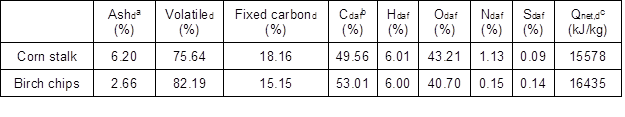a d: Dry basis. b daf: Dry, ash free basis. c Qnet, d: Net calorific value, dry basis.

RESULTS AND DISCUSSION

A TG curve and the corresponding DTG curve, which were obtained under the nitrogen atmosphere at the heating rate of 10 C/min, were taken as standards to analyze the biomass pyrolysis process (see Fig. 1).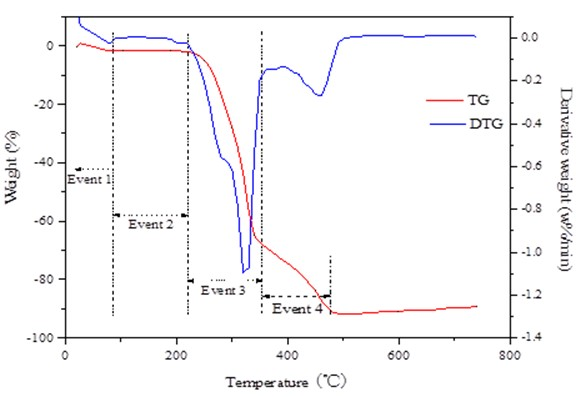Fig. 1. Pyrolysis of birch chips; β＝10 C/min

The thermal weight loss can be divided into four zones. The first zone is from the initial temperature to 90 C. With the increase of temperature, the moisture inside the birch chips will lessen, and a small peak will appear in the DTG curve. These features are characteristic of a drying stage. The second zone is from 90 to 220 C. The weight loss curve is almost flat, and a trace amount of weight loss occurs; the biomass changes into an amorphous solid (like glass), where no crystalline structures exist; such a result can be attributed to a slow process of depolymerization. The third zone is from 220 to 360 C. It corresponds to the pyrolysis process of hemicellulose and cellulose. Most of the weight loss occurs. The fourth zone is from 360 C to the final temperature. It is usually regarded as representing the decomposition process of lignin and cellulose. The process occurs slowly and will exceed the temperature range of the pyrolytic reaction (Lapuerta et al. 2004).

Thermogravimetric Analysis of Birch Chips and Corn Stalk Pyrolysis

Figure 2 is a comparison of the TG/DTG curves of birch chips at the specified rates of temperature rise under a nitrogen atmosphere and a carbon dioxide atmosphere. With the increase of the heating rate, the weight loss curve shifted to the high-temperature side, while the weight loss declined slightly. The peak value of the DTG curve shifted to the high-temperature side, exhibiting that under a situation with the same weight loss, the pyrolysis temperature required increases gradually. The influence of the heating rate was not as significant as the magnitude of these three heating rates. Therefore, the pyrolysis mechanisms were similar. In the Event 1 and 2 zones, the TG curves under these two carrier gas conditions almost overlapped each other. In comparison, in the Event 3 and 4 zones, the weight loss curves under these two carrier gas conditions differed slightly.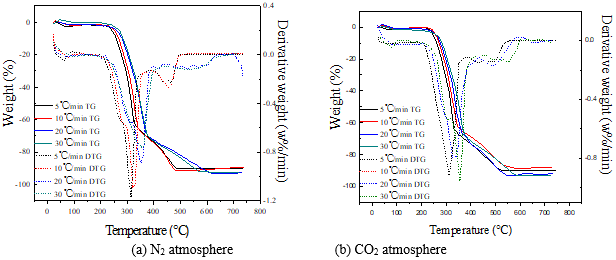Fig. 2. TG/DTG curves of birch chips pyrolysis under N2 and CO2 atmospheres

Figure 3 shows the comparison of the TG and DTG curves of corn stalk under different heating rate conditions and nitrogen and carbon dioxide atmospheres. The tendency of these curves was similar with that of the birch chips pyrolysis.

From Figs. 2 and 3, it looks like the two lower heating rates and the two higher heating rates form a pair. The reason is that at lower heating rates the pyrolysis process of hemicellulose tends to be completed before the pyrolysis process of lignin, but at higher heating rates, the pyrolysis processes of hemicellulose and lignin will overlap partly.

Figure 4 shows the TG curves of both birch chips and corn stalk that were obtained under a nitrogen atmosphere and a heating rate of 10 C/min. The figure reveals that the TG curves of birch chips and corn stalk almost overlapped with each other.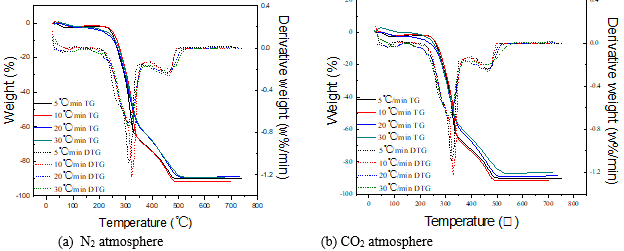Fig. 3. TG/DTG curves of corn stalk pyrolysis under N2 and CO2 atmospheres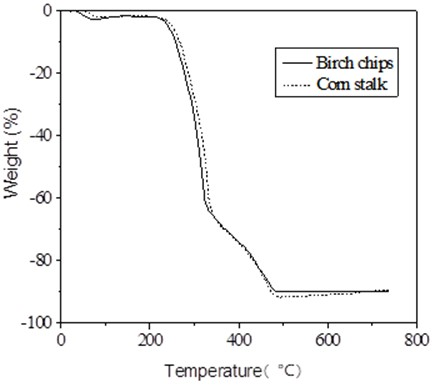Fig. 4. Comparison of different biomasses

Kinetic Parameters of Biomass Pyrolysis

The kinetic parameters can be confirmed by the data from the TG and DTG curves. With the adoption of an improved Coats-Redfern method and the application of 46 types of common kinetics mechanism functions, a mathematical analysis can be conducted. A reaction equation for thermal decomposition was set as:

BiomassSolid Remnant ＋ Gas.

The general form of the kinetic equation was as follows: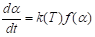(1)

According to the Arrhenius equation,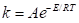(2)

and under the constant heating rate of programming,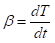(3)

where, is the constant of reaction rate in s-1is the pre-exponential factor in s-1β is the heating rate in C/min;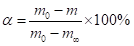is the change rate of reaction in % (m0 is the initial mass, m is the mass at t and m is the final mass.); is activation energy of reaction in kJ/mol; is the reaction temperature in K; and is the reaction order. So the equation becomes: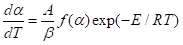(4)

After separating the variables from both sides and integrating, the equation becomes,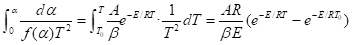(5)

which is the improved Coats-Redfern Integral Method. The general decomposition reaction is represented by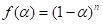. Taking the natural logarithm of both sides of the equation produces the following: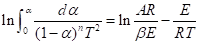(6)

Substituting a group of experimental data (,,=1, 2,…) into (5), yields the following: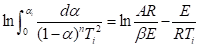，=1, 2,… (7)

Letting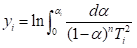, followed by using the iterative method, yields the following: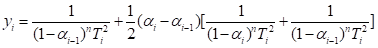(8)

where,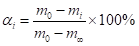Then, letting xi equal 1/Ti, and substituting it into Eq. 7,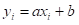(1, 2,…), where = –E/R (9) and b = ln (AR/E) (10)

allows the least squares method to be used. Simplifying the equation yields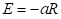and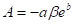. A plot of yi against xi should be a straight line with a slope –E/R since ln (AR/E) is nearly constant.

Another kinetic parameter can be calculated by applying the fundamental equation of activating reaction,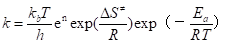(11)

where is the rate constant of reaction kinetics, kis the Boltzmann constant, is the Planck constant,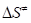is the activation entropy of reaction, and is the molecules of reaction. If it is assumed that it is a monomolecular reaction, is 1, and a correction factor of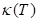(known as the penetration coefficient) is introduced, the following equation will apply: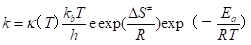(12)

Then it can be compared with the Arrhenius equation: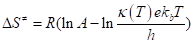(13)

The thermodynamic formulas apply,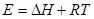， and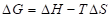(14)

where,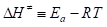and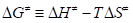. The enthalpy change, entropy change, and Gibbs free energy change that occurred from reagent to activated complex are respectively called activation enthalpy, activation entropy, and activation Gibbs free energy.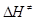is referred to as the activation of enthalpy of the reaction andis referred to as the activation free energy of the reaction.,,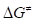at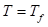(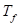is the maximum inflection point of TG curve) were calculated.is an important parameter as it is the demarcation point between two leading reaction stages in pyrolysis.

The spatial effect refers to an effect where the size of substituent groups or shapes gives rise to special tensions or resistances in the molecule. Stereo-hindrance effect can directly impact the reaction performance of a molecular compound and can also be the factor that impacts the activation of the reaction under most circumstances. P is used to represent the steric hindrance factor (Vlaev et al. 2003):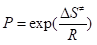(15)

The apparent activation energy of the fastest region of thermal weight loss, the frequency factor, the correlation coefficient, and the residual variance can all be calculated with the application of TG data recorded in the instrument, the adoption of an improved Coats-Redfern equation, 46 types of common kinetic mechanism function, and the least square and iterative methods. According to the maximum absolute value of the correlation coefficient, the minimum residual variance, and based on the fact that the activation energy of common chemical reaction is within the scope of (40 to 400) kJ/mol, the chemical reaction of activation energy, which is below 40 kJ/mol, is fast enough in chemical reaction velocity that it will be accomplished instantly. The chemical reaction of activation energy, which is above 400 kJ/mol, is extremely slow for a chemical reaction rate. The high value may be understood as an indication that the system does not undergo a chemical reaction to an important extent (Garcia-Bacaicoa et al. 2008). The differential form of the reaction mechanism function is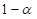, and its corresponding integral form of the mechanism function is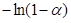. Therefore, the reaction is a first-order reaction.

Table 2, in Event 3, shows a difference between the apparent activation energy under the nitrogen atmosphere and the carbon dioxide atmosphere. It corresponds to the thermogravimetric curve obtained in the experiment under the nitrogen and carbon dioxide atmospheres. The apparent activation energy values of the two biomass samples in Event 3 do not differ greatly, indicating that the reaction mechanisms of these two atmospheres are similar. The apparent activation energy value in Event 4 is fairly small, indicating that the activation energy value contained in the volatile matter is fairly small. This can be attributed to the fact that the sample’s activation energy contained is low.

To better understand the characteristics of biomass pyrolysis kinetics, the primary parameter and activation characteristic value of biomass pyrolysis are connected. With regards to a monomolecular reaction, when the activated complex structures of transition states are similar to the reactant, the pre-exponential factor has a “normal” value that is about 1013 s-1. When< 0, the pre-exponential factor of many monomolecular reactions is between 109 and 1011 s-1. However, the pre-exponential factor obtained from the experiment is smaller as a cyclic structure is formed and several rotational degrees of freedom have been translated into vibration degree of freedom. Comparing the steric hindrance factor, P, in Table 2, the steric hindrance factor of Event 3 is significantly smaller than that of the Event 4. Therefore, Event 3 is regarded as a faster reaction.

CONCLUSIONS

1. Biomass pyrolysis can be divided into two leading reaction zones, Event 3 and Event 4. Event 3 is oriented by cellulose and hemicellulose. Event 4 is oriented by lignin and cellulose. The inflection point temperatures of the two zones are the demarcation points of the leading reaction stages in the process of biomass pyrolysis.
2. The thermogravimetric curves of the two biomasses under carbon dioxide and nitrogen atmosphere are roughly similar, indicating that both of the gases are suitable atmospheres for biomass pyrolysis.
3. The differential form of the reaction mechanism function isand its corresponding integral form of the mechanism function is.
4. It is possible to use the activation entropy to represent the pre-exponential factor and to use the steric hindrance factor to predict the reaction rate.

REFERENCES CITED

Basu, P. (2013). Biomass Gasification, Pyrolysis, and Torrefaction: Practical Design and Theory, Elsevier, London.

Blasi, C. D. (2008). “Modeling chemical and physical processes of wood and biomass pyrolysis,” Progress in Energy and Combustion Science 34, 47-90. DOI: 10.1016/j.pecs.2006.12.001

Cuevas, A., Reinoso, C., and Lamas, J. (1994). “Advance and developments at the union fenosa pyrolysis plant,” in: Proc. 8th European Conference on Biomass, 3-5 October 1994, Vienna, Italy, pp. 1506-1512.

Demirbas, A. (2000). “Mechanisms of liquefaction and pyrolysis reactions of biomass,” Energy Conversion and Management 41(6), 633-646. DOI: 10.1016/S0196-8904(99)00130-2

Garcia-Bacaicoa, P., Mastral, J. F., Ceamanos, J., Berrueco, C., and Serrano, S. (2008). “Gasification of biomass / high density polyethylene mixtures in a downdraft gasifier,” Bioresource Technology 99(13), 5485-5491. DOI: 10.1016/j.biortech.2007.11.003

Lapuerta, M., Hernandez, J. J., and Rodriguez, J. (2004). “Kinetics of devolatilisation of forestry wastes from thermogravimetric analysis,” Biomass & Bioenergy 27(4), 385-391. DOI: 10.1016/j.biombioe.2003.11.010

Maia, A. A. D., and de Morais, L. C. (2016). “Kinetic parameters of red pepper waste as biomass to solid biofuel,” Bioresource Technology 204, 157-163. DOI: 10.1016/j.biortech.2015.12.055

Moriana, R., Vilaplana, F., Karlsson, S., and Ribes, A. (2014). “Correlation of chemical, structural and thermal properties of natural fibres for their sustainable exploitation,” Carbohydrate Polymers 112, 422-431. DOI: 10.1016/j.carbpol.2014.06.009

Ranzi, E., Cuoci, A., Faravelli, T., Frassoldati, A., Migliavacca, G., Pierucci, S., and Sommariva, S. (2008). “Chemical kinetics of biomass pyrolysis,” Energy and Fuels 22(6), 4292-4300. DOI: 10.1021/ef800551t

Scott, D. S., Piskorz, J., and Graham, R. G. (1987). “The effect of temperature on liquid product composition from the fast pyrolysis of cellulose,” American Chemical Society, Division of Fuel Chemistry, 5 April 1987 (United States), 32(CONF-870410-).

Sun, L., and Zhang, X. (2013). Principle and Technology of Biomass Pyrolysis and Gasification, Chemical Industry Press, Beijing, China.

Vlaev, L. T., Markovska, I. G., and Lyubchev, L. A. (2003). “Non-isothermal kinetics of pyrolysis of rice husk,” Thermochimica Acta 406(1-2), 1-7. DOI: 10.1016/S0040-6031(03)00222-3

Zellagui, S., Schönnenbeck, C., Zouaoui-Mahzoul, N., Leyssens, G., Authier, O., Thunin, E., Porcheron, L., and Brilhac, J.-F. (2016). “Pyrolysis of coal and woody biomass under N2 and CO2atmospheres using a drop tube furnace – experimental study and kinetic modeling,” Fuel Processing Technology 148, 99-109. DOI: 10.1016/j.fuproc.2016.02.007

Article submitted: June 13, 2016; Peer review completed: August 7, 2016; Revised version received and accepted: August 8, 2016; Published: August 24, 2016.

DOI: 10.15376/biores.11.4.8548-8557

Table 2. Kinetic Parameters of Biomass Pyrolysis